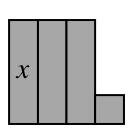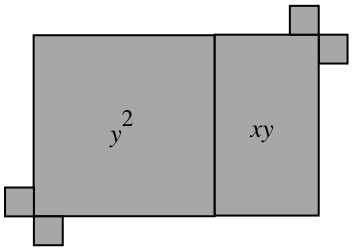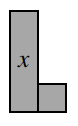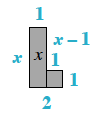### Home > INT2 > Chapter 1 > Lesson 1.2.3 > Problem1-50

1-50.

For each of the shapes formed by algebra tiles below:

• Sketch and label the shape on your paper and write an expression that represents the perimeter.

• Simplify your perimeter expression as much as possible.

1.1.Start by labeling the sides.$x+1+(x-1)+1+1+2$
Combine like terms.

$2x + 4$